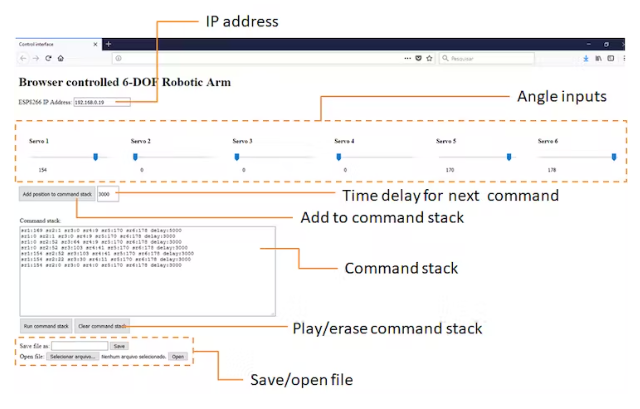• 正文
• 相关推荐
• 电子产业图谱

# 基于ESP8266的Wi-Fi控制机械臂

2022/10/14352扫码加入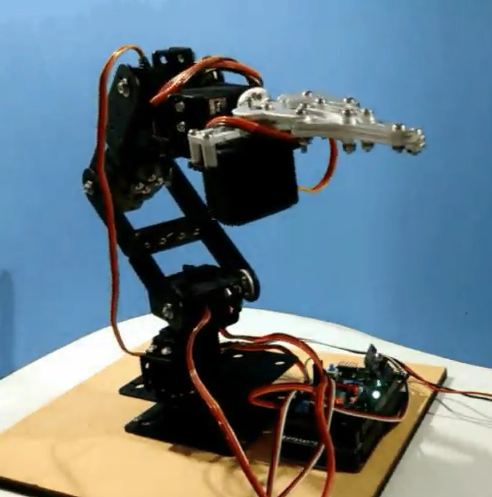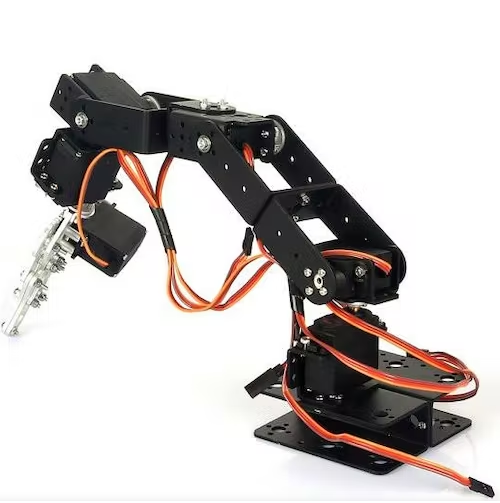• 焊锡铁丝。为了将Nunchuk连接到Arduino上，我必须在Nunchuk的电线上焊接一些端子;
• 收缩管。一些缩管片被用来更好地隔离导体;
• 螺丝刀。该结构是用一些螺栓和螺母安装的;
• 六轴机械台式机械臂
• 12V电源(2A或以上);
• ESP8266-01
• 跳线(5线);
• Arduino Mega

Sain智能6轴机械桌面臂已经配备了以下组件:

• Arduino Mega 2560 R3
• 控制板屏蔽
• NRF24L01+无线收发模块
• MPU6050三轴陀螺仪及三轴加速度计
• 71 × m3 × 8螺钉
• 47 × M3螺母
• 2 * U支架
• 5倍伺服支架
• 4 x 9公斤伺服
• 2 x 20公斤伺服
• 6 x金属伺服托盘
• 3 × U支架
• 21x直角支架
• 3 ×法兰轴承
• 1 x机械手掌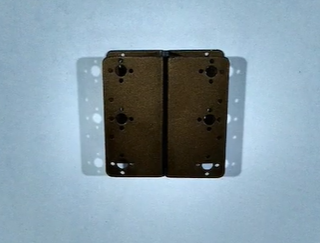• 第一个要组装的部件是机器人的底座。它由两个U形支架组成，用四个M3螺栓和螺母背对背连接，如图所示
• 伺服电机安装垂直于底座，使用伺服支架。这个剖面使用四个M3螺栓和螺母连接到底座上，如图所示。伺服#1放在它的顶部，并使用四个M3螺栓和螺母连接。一个圆形的金属喇叭附在伺服轴上。
• 另一个伺服支架安装垂直于前一个。它连接到伺服1号喇叭使用四个M3螺栓。伺服#2安装有四个M3螺栓和螺母，也使用圆形金属喇叭。一个U型支架，然后连接到喇叭使用四个螺栓。注意，在伺服轴的正侧使用了一个M3螺栓。它使结构稳定。一个轴承安装在这个螺栓上，并使用另一个M3螺母锁定在位置上。这样U型支架紧密地连接到伺服#2中心轴上。
• 另一个U型支架使用4颗M3螺栓和螺母安装。在另一端，安装伺服#3，使用一个圆形金属喇叭和四个螺栓。伺服支架与伺服电机连接，用一些螺栓和螺母将L型型材与伺服支架连接。注意，另一个轴承是用对伺服轴，如前所述。
• 还有一个U型支架使用一组M3螺栓和螺母连接到L型型材上。类似于前面的步骤，伺服#4是安装到U支架使用四个螺栓。另一个伺服支架与伺服连接。
• 第五个伺服与伺服4号垂直连接，使用另一个伺服支架，安装使用四个M3螺栓和螺母。
• 夹持器连接到伺服#5轴上。在它的顶部，伺服#6连接使用一些螺栓，螺母和一个金属喇叭。夹持器有一些齿轮，这将把伺服的旋转变成夹持器的线性运动。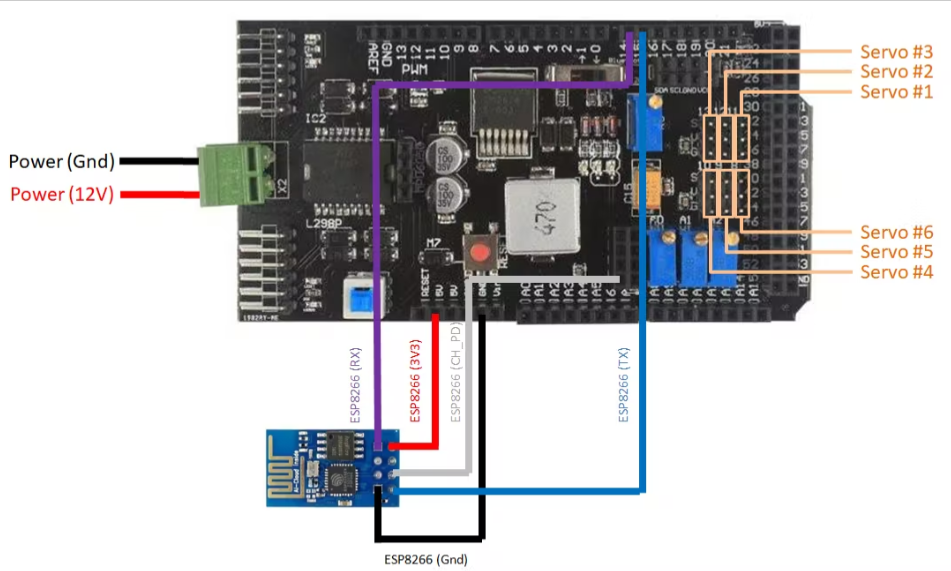ESP8266:

• Arduino Mega Pin 14(在屏蔽上)
• Arduino Mega Pin 15(在屏蔽上)
• ESP8266 Vcc => Ardino Mega Pin 3V3(在盾牌上)
• ESP8266 Gnd => Arduino Mega Pin Gnd(在屏蔽上)
• ESP8266 CH_PD => Arduino Mega Pin 3V3(在屏蔽上，在为24L01模块预留的连接器中)

• 控制屏蔽端子11 =>伺服#1
• 控制屏蔽端子12 =>伺服#2
• 控制屏蔽端子13 =>伺服#3
• 控制屏蔽端子8 =>伺服#4
• 控制屏蔽端子9 =>伺服#5
• 控制屏蔽端子10 =>伺服#6

• Arduino Pin 11 =>伺服#1 (Sgn)
• Arduino Pin 12 =>伺服#2 (Sgn)
• Arduino Pin 13 => 3伺服(Sgn)
• Arduino Pin 8 =>伺服#4 (Sgn)
• Arduino Pin 9 =>伺服#5 (Sgn)
• Arduino Pin 10 =>伺服#6 (Sgn)
• Arduino Gnd => Servos Gnd
• 6V电源=>伺服Vcc

1. 下载并安装Arduino IDE最新版本您可以在Arduino的网站上找到Windows, Linux或MAC OSX的最新版本:https://www.arduino.cc/en/main/software

Arduino与with ESP8266模块之间的通信没有使用额外的库。请检查您ESP8266的波特率，并在代码中正确设置。

Servo_speed变量定义所有伺服的运动速度。如果你想要一个特定的伺服移动更快，增加它的值。

//Include libraries
#include <Servo.h>
//define variables
#define DEBUG true //display ESP8266 messages on Serial Monitor
#define SERV1 8    //servo 1 on digital port 8
#define SERV2 9    //servo 2 on digital port 9
#define SERV3 10   //servo 3 on digital port 10
#define SERV4 11   //servo 4 on digital port 11
#define SERV5 12   //servo 5 on digital port 12
#define SERV6 13   //servo 6 on digital port 13
Servo s1; //servo 1
Servo s2; //servo 2
Servo s3; //servo 3
Servo s4; //servo 4
Servo s5; //servo 5
Servo s6; //servo 6
//define starting angle for each servo
//choose a safe position to start from
//it will try to move instantaniously to that position when powered up!
//those angles will depend on the angle of each servo during the assemble
int angle1 = 90; //servo 1 current angle
int angle2 = 30; //servo 2 current angle
int angle3 = 0;  //servo 3 current angle
int angle4 = 90; //servo 4 current angle
int angle5 = 90; //servo 5 current angle
int angle6 = 45; //servo 6 current angle
int servo_speed = 6; //speed of the servos
int angle1sp = 90; //servo 1 set point
int angle2sp = 30; //servo 2 set point
int angle3sp = 0;  //servo 3 set point
int angle4sp = 90; //servo 4 set point
int angle5sp = 90; //servo 5 set point
int angle6sp = 45; //servo 6 set point
boolean display_angles = true; //boolean used to update the angle of each servo on Serial Monitor

AT+CIFSR命令用于显示归属于ESP8266的IP地址。稍后将在控件接口上使用它。然后在端口80启动web服务器。这样，ESP8266将能够接收到该IP地址和端口的消息。

//SETUP
void setup() {
//attach each servo to a pin and start its position
s1.attach(SERV1);
s1.write(angle1);
s2.attach(SERV2);
s2.write(angle2);
s3.attach(SERV3);
s3.write(angle3);
s4.attach(SERV4);
s4.write(angle4);
s5.attach(SERV5);
s5.write(angle5);
s6.attach(SERV6);
s6.write(angle6);
//start serial communication
Serial.begin(9600);
Serial.println("Connecting...");
Serial3.begin(9600);
//Wi-Fi connection
sendData("AT+RSTrn", 2000, DEBUG); //reset module
sendData("AT+CWMODE=1rn", 1000, DEBUG); //set station mode
sendData("AT+CWJAP="XXXXX","YYYYY"rn", 2000, DEBUG);   //connect wifi network
while(!Serial3.find("OK")) { //wait for connection
}
sendData("AT+CIFSRrn", 1000, DEBUG); //show IP address
sendData("AT+CIPMUX=1rn", 1000, DEBUG); //allow multiple connections
sendData("AT+CIPSERVER=1,80rn", 1000, DEBUG); // start web server on port 80
}

void loop() {
if (Serial3.available())  //check if there is data available on ESP8266
{
if (Serial3.find("+IPD,")) //if there is a new command
{
String msg;
Serial3.find("?"); //run cursor until command is found
String command = msg.substring(0, 3); //command is informed in the first 3 characters. "srs" = command to move the six servos
String valueStr1 = msg.substring(4, 7);   //next 3 characters inform the desired angle
String valueStr2 = msg.substring(8,11);   //next 3 characters inform the desired angle
String valueStr3 = msg.substring(12,15);   //next 3 characters inform the desired angle
String valueStr4 = msg.substring(16,19);   //next 3 characters inform the desired angle
String valueStr5 = msg.substring(20,23);   //next 3 characters inform the desired angle
String valueStr6 = msg.substring(24,27);   //next 3 characters inform the desired angle
int angle1sp = valueStr1.toInt();         //convert to integer
int angle2sp = valueStr2.toInt();         //convert to integer
int angle3sp = valueStr3.toInt();         //convert to integer
int angle4sp = valueStr4.toInt();         //convert to integer
int angle5sp = valueStr5.toInt();         //convert to integer
int angle6sp = valueStr6.toInt();         //convert to integer

if (angle1 > angle1sp) {
angle1 -= 1;
s1.write(angle1);
}
if (angle1 < angle1sp) {
angle1 += 1;
s1.write(angle1);
}
.
.
.
delay(100/servo_speed);

“添加位置到命令堆栈”按钮允许用户在文本框中存储手臂在命令堆栈上的当前位置。通过这种方式，可以存储位置序列，每个移动之间具有可配置的延迟。

Html代码注释:

Html文件分为头部和主体部分。头部只显示一些元数据，并指示将在该文件上运行哪些javascript文件。

<html xmlns="http://www.w3.org/1999/xhtml">
<meta http-equiv="Content-Type" content="text/html; charset=UTF-8" />
<meta name="author" content="Igor Fonseca Albuquerque">
<title>Control interface</title>
<script src="jquery.js"></script>
<script src="myscript1.js"></script>

<div style="margin: 0; width:500px; height:80px;">
<form name="myform2" action="" method="GET">
<input type="text" name="inputbox" value="192.168.0.9">
</form>
</div>

<th>
<p style="margin: 0; position: relative; top: 0px; left: 0px; width:100px; height:20px;"> Servo 1 </p>
<input type="range" id="s1Range" min="0" max="180" value="90" onmouseup="moveSrs(s1Range.value, s2Range.value, s3Range.value, s4Range.value, s5Range.value, s6Range.value)" onchange="updateTextInput1(this.value)" style="margin: 0; position: relative; top: 10px; left: 0px; width:200px; height:40px;" />
<input type="text" id="s1textInput" value="90" style="margin: 0; position: relative; top: 10px; left: -10px; border: none;">
</th>

<input type="button" id="button1" value="Add position to command stack" onClick="addCommand(s1textInput.value, s2textInput.value, s3textInput.value, s4textInput.value, s5textInput.value, s6textInput.value, delayValue.value)" style="position: relative; top:60px; height:40px;">
<input type="text" id="delayValue" value="1000" style="position: relative; top:60px; height:35px; width:50px;">

<table style="margin: 0; position: relative; top: 80px; left: 0px; width:100%; height:80px;">
<tr><td>Command stack:</td></tr>
<tr>
<td>
<textarea id="inputTextToSave" cols="80" rows="14"></textarea>
</td>
</tr>
<tr>
<td>
<button onclick="runCommands(inputTextToSave.value)" style="height:40px;">Run command stack</button>
<button onclick="clearCommand()" style="height:40px;">Clear command stack</button>
<p>
</td>
</tr><br>

Javascript代码注释:

html接口调用两个Javascript文件:jquery.js和myscript.js。在myscript.js中，我设计了自己的函数来与机器人交互。

function moveSrs(angle1, angle2, angle3, angle4, angle5, angle6)
{
TextVar = myform2.inputbox.value;
ArduinoVar = "http://" + TextVar + ":80";

if (angle1 < 100) {
angle1 = "0" + angle1;
}
if (angle1 < 10) {
angle1 = "0" + angle1;
}
.
.
.
//send command
\$.wait(5000).then(\$.get( ArduinoVar, { "srs": angle1 + " " + angle2 + " " + angle3 + " " + angle4 + " " + angle5 + " " + angle6 }, function(data){alert("data:" + data);}))    ;
{Connection: close};

}

//update text under scroll bars
function updateTextInput1(val) {
document.getElementById('s1textInput').value=val;
}

function addCommand(val1, val2, val3, val4, val5, val6, val7) {
document.getElementById('inputTextToSave').value = document.getElementById('inputTextToSave').value + "sr1:" + val1 + " sr2:" + val2 + " sr3:" + val3 + " sr4:" + val4 + " sr5:" + val5 + " sr6:" + val6 + " delay:" + val7 + String.fromCharCode(13, 10);
}
//clear command stack
function clearCommand() {
document.getElementById('inputTextToSave').value = "";
}

runCommands()是处理命令堆栈的地方。它每次在线读取并将其拆分以获得该命令行中每个伺服的角度。我注意到使用了一个setTimout函数。时间变量'k'在每个命令行上按给定的时间(延迟值)递增。使用setTimout函数，一个新的命令只在给定的时间过后发送，并且ESP8266不会同时收到很多消息。否则，它的缓冲区将被超过，可能会崩溃。

//run command stack
function runCommands(val) {
var k = 0;
step = 0;
var commands = val.split("n"),i;
for (i = 0; i < commands.length - 1; i++) {
setTimeout(function(data){
var angle1 = commands[step].split(" ");
angle1 = angle1.split(":");
var angle2 = commands[step].split(" ");
angle2 = angle2.split(":");
var angle3 = commands[step].split(" ");
angle3 = angle3.split(":");
var angle4 = commands[step].split(" ");
angle4 = angle4.split(":");
var angle5 = commands[step].split(" ");
angle5 = angle5.split(":");
var angle6 = commands[step].split(" ");
angle6 = angle6.split(":");
moveSrs(angle1, angle2, angle3, angle4, angle5, angle6);
step+=1;
},k);

var tempo = commands[step].split(" ");
tempo = tempo.split(":");
k += parseInt(tempo);
}
}